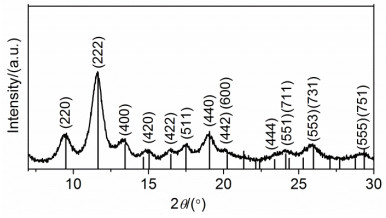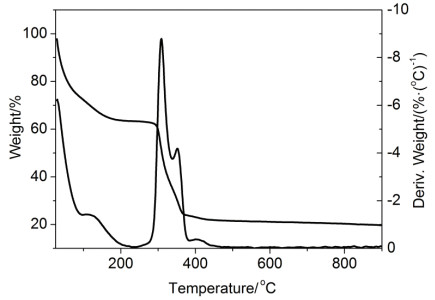## Synthesis of Metal-Organic Framework Nanocrystals and Research on Its Adsorption of Dye,, , , , , ,,

 基金资助: 国家精品课程广东省精品课程国家自然科学基金.  21571193稀土资源利用国家重点实验室开放课题基金.  RERU2013012

 Fund supported: 国家精品课程广东省精品课程国家自然科学基金.  21571193稀土资源利用国家重点实验室开放课题基金.  RERU2013012Abstract

A research-type comprehensive experiment, which involves synthesis, characterization and application study of metal-organic framework (MOFs) nanocrystals, was introduced. A creative strategy was used to prepare metal-organic framework nanocrystals with smallest particle size amongst published MOFs. The essential characterization methods for nanomaterial and adsorption kinetics study were presented so that the students can get knowledge of the research in the nanomaterial field. The experiment system can serve to stimulate students' interest in scientific research and innovation consciousness and train students' chemical thoughts by analysis of the results from different react conditions.

Keywords： Metal-organic framework nanocrystals ; Ultrasonic synthesis ; Kinetics of dye adsorption ; Experimental design

QIAO Zhengping, YIN Mingda, XU Xianfang, HUANG Huazhen, YAN Sujun, PENG Huijuan, MAO Zongwan. Synthesis of Metal-Organic Framework Nanocrystals and Research on Its Adsorption of Dye. University Chemistry[J], 2018, 33(9): 75-81 doi:10.3866/PKU.DXHX201802015

(1)产品易合成、产率高，反应温度、时间影响不大，实验平行性好；

(2)产品形貌和粒径受反应物种类、浓度、超声波合成仪功率、溶剂、表面活性剂影响，可让学生广泛设计新的实验方案，探索最佳反应条件，分析反应机理；

(3)产品粒径、染料分子结构影响吸附性能，通过吸附动力学研究，从微观角度解释吸附机理。

(4)涉及多种实验技术：X射线粉末衍射法、拉曼光谱法、透射电镜法、紫外-可见吸收光谱。

### 1.1 主要仪器和试剂

 仪器 型号 厂家 超声波清洗机 AS3120A 天津Auto Science仪器有限公司 X射线粉末衍射仪 D8 ADVANCE03030502 德国Bruker公司 200 kV透射电镜 JEM-2010HR 日本电子株式会社JEOL 激光显微拉曼光谱仪 Renishaw inVia 英国Renishaw公司 热重分析仪 TG-209 德国Netzsch公司 Zeta电位仪 ZetaPALS 美国Brookhaven公司 紫外-可见分光光度计 UV-3150 日本岛津公司

#### 1.2.2 染料吸附性能测试

1)绘制染料溶液UV-Vis吸收工作曲线。

2)染料吸附性能研究。

### 图1### 图2### 图3### 图4##### 1.3.6 吸附动力学分析

${Q_{\rm{t}}} = \frac{{({\mathit{c}_0} - {\mathit{c}_{\rm{t}}})\mathit{V}{\mathit{M}_{\rm{r}}}}}{m}$

 动力学模型 微分形式 积分形式 准一级动力学模型 $\frac{{{\rm{d}}{\mathit{Q}_{\rm{t}}}}}{{{\rm{d}}\mathit{t}}} = {\mathit{k}_{\rm{1}}}({Q_{\rm{e}}} - {\mathit{Q}_{\rm{t}}})$ ln(Qe - Qt) = lnQe - k1t 准二级动力学模型 $\frac{{{\rm{d}}{\mathit{Q}_{\rm{t}}}}}{{{\rm{d}}\mathit{t}}} = {\mathit{k}_2}{({Q_{\rm{e}}} - {\mathit{Q}_{\rm{t}}})^2}$ $\frac{\mathit{t}}{{{Q_{\rm{t}}}}} = \frac{\mathit{t}}{{{Q_{\rm{e}}}}} + \frac{\mathit{t}}{{{k_{\rm{2}}}Q_{\rm{e}}^2}}$

*k1k2分别为准一级、准二级动力学速率常数，Qe为平衡时的吸附量

 动力学模型 微分形式 线性形式 Langmuir吸附等温式模型 ${Q_{\rm{e}}} = \frac{{{Q_{\max }}{K_{\rm{L}}}{\mathit{c}_{\rm{e}}}}}{{1 + {K_{\rm{L}}}{\mathit{c}_{\rm{e}}}}}$ $\frac{{{\mathit{c}_{\rm{e}}}}}{{{Q_{\rm{e}}}}} = \frac{{{\mathit{c}_{\rm{e}}}}}{{{Q_{\max }}}} + \frac{1}{{{\mathit{Q}_{{\rm{max}}}}{K_{\rm{L}}}}}$ Freundlich吸附等温式模型 Qe = KFce1/n $\ln {Q_{\rm{e}}} = \frac{1}{n}\ln {\mathit{c}_{\rm{e}}} + \ln {\mathit{K}_{\rm{F}}}$

*ce为平衡时染料的浓度，Qe为平衡吸附量，Qmax为材料的理论最大吸附量，
KLKF分别为Langmuir和Freundlich常数

## 参考文献 原文顺序 文献年度倒序 文中引用次数倒序 被引期刊影响因子

Qi Y. ; He C. T. ; Lin J. ; Lin S. ; Liu J. ; Huang J. ; Xue W. ; Yu G. ; Chao H. Y.s ; Tong Y. ; Qiao Z. Nano Res 2017, 10 (11), 3621.

YakovenkoA. A. ; Reibenspies J. H.s ; Bhuvanesh N. ; Zhou H. C. J. Appl. Cryst 2013, 46, 346.

PrestipinoC. ; Regli L. ; Vitillo J. G. ; Bonino F. ; Damin A. ; Lamberti C. ; Zecchina A. ; Solari P. L. ; Kongshaug K. O. ; Bordiga S. Chem. Mater 2006, 18 (1337)

/

 〈〉